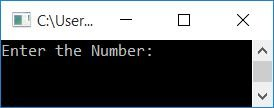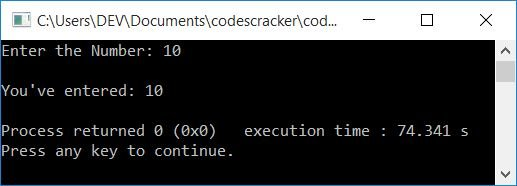# C++ Program to Print an Integer

In this article, you will learn and get code to print an integer value using a C++ program. Here is the list of programs for printing an integer (number):

## Print an integer in C++

In C++ programming, simply put the variable that holds the value after cout to print any integer or number on output.

`cout<<num;`

Whatever the value of num is, it gets printed on the output. Here is the complete program for printing an integer value along with some string (or message) before it.

```#include<iostream>
using namespace std;
int main()
{
int num=10;
cout<<"The Value of 'num' is "<<num;
cout<<endl;
return 0;
}```

This program was built and runs under the Code::Blocks IDE. Here is its sample output:You can replace the following statement:

`cout<<"The Value of 'num' is "<<num;`

with

`cout<<num;`

to print only 10 on the output, without any extra things.

### Print an integer (number) entered by the user

This program allows the user to enter the number. When the user enters a number, say 10, it gets stored in num. And print the value of num on the output in a similar way as already discussed in the previous program.

```#include<iostream>
using namespace std;
int main()
{
int num;
cout<<"Enter the Number: ";
cin>>num;
cout<<"\nYou've entered: "<<num;
cout<<endl;
return 0;
}```

The snapshot given below shows the initial output produced by this program:Now supply the input as a number, say 10, and then press ENTER to print the entered number back on the output screen as shown in the sample output given below:#### The same program in different languages

C++ Quiz

« Previous Program Next Program »